Next: 5.3.2.2 Simulation of the Output Characteristics Up: 5.3.2 Fitting Procedure Previous: 5.3.2 Fitting Procedure

5.3.2.1 Simulation of the Transfer Characteristics

It is useful to start the fitting procedure with the threshold voltage VT. Regarding the simulation this is mainly based on the solution of Poisson's equation and thus can be considered as very reliable. Regarding device parameters VT is mainly determined by the composition of the epitaxial layers, the effective barrier height of the gate Schottky contact FB, the location and concentration of the doping in the supply NDS and in the buffer layer NDB as well as the gate to channel separation dGC.

The indium and aluminum contents of the layers can be controlled very precisely by MBE growth in respect to their influence on the device characteristics, therefore the nominal values can be taken for the simulation. FB is dependent on the applied technology which produces the physical contact interface. It can be determined by measurements. A typical value and range over one wafer which can be also considered as the fitting range is 650 mV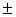25 mV. The location of the doping concentration is controlled precisely by MBE. Depending on the epitaxial growth and the following HEMT process some segregation and out-diffusion effects from a delta doping should be considered. However, the doping concentration is subject to significant uncertainties. A typical value and uncertainty range for delta doping is about 60 %10 % activation of the impurities incorporated in the crystal. The uncertainty in the activation of homogeneously doped layers is about5 %.

A change of both the parameters FB and NDB leads basically to a parallel shift of the transfer characteristics. Therefore they are rather uncritical parameters despite their uncertainties. The value of NDS is somewhat more critical because it not only shifts VT but also has a moderate impact on gm. Thus, the nominal value NDS has to be taken to fit VT and then possibly has to be changed when other characteristics are fitted.

Fitting the transfer characteristics for VGS > VT both the carrier concentrations and the velocities have to be simulated. As described in Chapter 2 the most important electron transport region is the channel. As described in Section 4.3.2 the electron velocity in MINIMOS­NT is determined by the mobility and the driving force. In the HD case the reduction of the mobility in the high field regime is modeled by the electron temperature as shown in (46). Therefore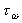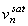, and b are fitting parameters for the electron velocity. The InGaAs saturation velocity vsat of manufactured devices can be estimated by a delay time analysis which is subject to an error of about 20% . Further investigations will not use this method as it does not distinguish between the velocity overshoot and vsat therefore only reveals an average velocity.

In  Figure 5.20 the electron velocity distribution in the channel for vsat = 1.1 * 105 ms­1, tw = 0.18 ps, and b = 0.9 at VDS = 2.0 V and VGS = 0.5 V is shown by the bold line. The curve with the triangles indicates a simulation were vsat is changed to 1.0 * 105 ms­1 thus also the peak velocity is reduced. A similar change is obtained for a reduction of b to 0.8 indicated by the squared symbols. This shows that vsat and b cannot be separated in this way. So far it is not clear whether the parameter b is necessary or not. Without the parameter b (i. e. b = 1) an extremely low vsat of about 0.9 * 105 ms-1 would be necessary to fit the measured results. The change of tw to 0.25 ps indicated by the triangles shows that this has no influence on the peak velocity but on the distribution before and after the peak.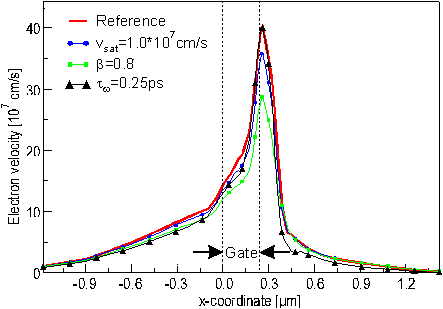Figure 5.20 Electron velocities in the channel at VDS = 2.0 V and VGS = 0.5 V. The bold line indicates a reference simulation with vsat = 1.1 * 105 ms-1, b = 0.9, and tw = 0.18 ps. In the simulation indicated by symbols one parameter is changed.

The electric current is the sum of electrons moving with different velocities undergoing various scattering events. To calculate the current flow with DD or HD models as described in Section 4.1 all these electrons and events are treated by average values. To develop the DD and HD models the electron distribution is assumed to be known a priori. Throughout this work Boltzmann's statistics are used. This is different in particle based methods such as Monte Carlo (MC) simulations were the microscopic scattering processes are described by given scattering probabilities. This way single electrons moving in different energy states are considered separately. The distribution in energy is a simulation result rather than known before. Figure 5.21 shows the velocities of electrons in the G and the X valley as well as the average electron velocity in the channel obtained by MC simulations [60, 61]. The HD simulation which was already shown in  Figure 5.20 is indicated by the bold line with circles. Both velocity distributions obtained by the two fundamentally different approaches show the same principle characteristics. The electron velocity exceeds vsat already at the source side of the gate, reaches its peak at the drain end, and is about a factor of three larger than vsat.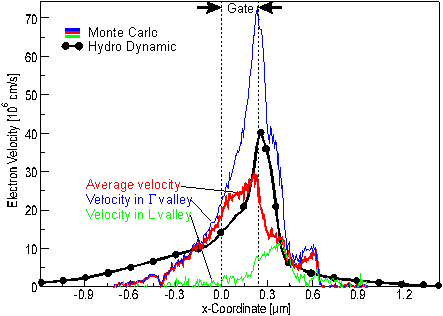Figure 5.21 Electron velocities in the channel calculated by Monte Carlo and mixed DD/HD simulations at VDS = 2.0 V and VGS = 0.5 V.

The peak velocity revealed by the HD simulation is higher than that of the average velocity obtained by the MC simulations. Publications of other MC simulations reported average velocities of similar HEMTs up to 6*107cm/s . A significant reduction in the peak velocity of the HD simulation would reveal a lower average velocity along the channel than predicted by the MC simulation. Although the characteristics calculated by the two different methods agree quite well in principle the differences in the details ask for further investigations on both approaches.

In the following the parameters for the HD model which resulted in the bold line of  Figure 5.21 will be used. To predict the magnitude of gm max and VGS at which it is reached not only VT and the current in the channel have to be modeled but also the saturation of the transfer characteristics due to real space transfer. This is mainly determined by the interface model as described in Section 4.2.1. The most important parameters are the energy relaxation time tw governing the electron temperature distribution and the parameters Bi of the exponential function in (34) which model the reduction of the barrier height due to tunneling.

In  Figure 5.22 simulated transfer characteristics are shown. The bold line with circles represents the simulation with tw = 0.18 ps and B1 = B2 = 1.0, Ei>2 = 0. If tw is increased to 0.25 ps as indicated by the squares the real space transfer is increased and thus ID is reduced stronger. A similar change can be observed when tunneling is enhanced by increasing B3 to 1.0 as indicated by the triangles. This also suggests that only the first factors of the Tailor series in (34) need to be taken to describe the reduction of DEC due to tunneling.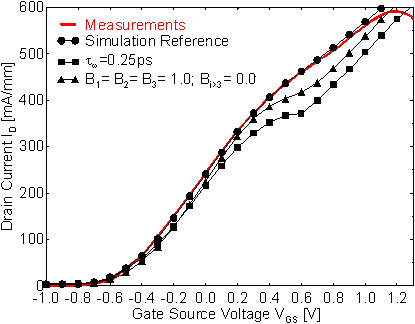Figure 5.22 Simulated transfer characteristics with different parameters governing. A change in the tunnel coefficients Bi has a similar effect on the transfer characteristics than a change in the tw of the HD model in the channel.

Using these two parameters tw and Bi the interface model is able to reproduce the transfer characteristics very well. But it must be pointed out that the model in its present state is not capable to predict changes in the saturation of the transfer characteristics due to changes in the band gap discontinuity such as a change in the indium content. A physical reason for this was discussed in Section 4.2.1.

The last important part of the transfer characteristics to be fitted is the parasitic MESFET region. It is basically related to the transport model used in the supply layer as described in Section 5.2. As shown a HD model in the AlGaAs supply layer is necessary to model the second local maximum in gm. The quantities vsat, b, and tw for AlGaAs are used as the fitting parameters. As discussed before there are still large discrepancies between the electron velocities obtained by HD and MC simulations. For a certain current density both the electron concentration and velocity are not known exactly, thus the product n*v is not unique.

Electron concentration and velocity can be separated by considering both the ID(VGS) and the CG(VGS) characteristics. An increase in carrier concentrations leads to an increase in both ID and CG but an increase in carrier velocity leads to an increase in ID and to a decrease in CG. Therefore a simultaneous fit of both gate capacitance and current characteristics is needed.

In  Figure 5.23 the gate capacitance extracted from S­parameter measurements of HEMTref is shown along with simulated CG curves. The procedure to obtain data which can be compared was described in Section 3.3. Also the corresponding measured and simulated values of gm are depicted which correspond to the data in  Figure 5.11 and  Figure 5.14.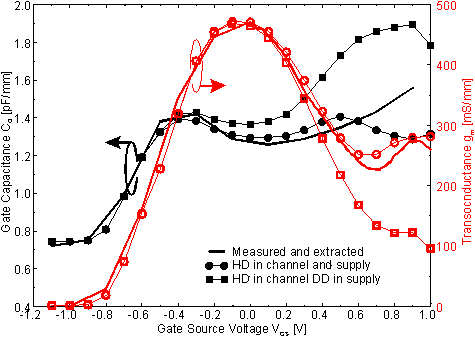Figure 5.23 Gate capacitance extracted from S­parameter measurements and mixed DD/HD simulations using a quasi static approximation at VDS = 2.0 V. An increase in electron velocity reduces CG but increases ID. This way the electron velocity can be separated from the electron concentration.

As a previous result DD transport in the supply layer revealed too low ID for VGS > 0.2 V. This is due to the relatively low velocity of electrons in the supply layer. Whereas for VGS < 0.2 V no difference in ID could be observed ( Figure5.11) and only a minor difference occurred in the transconductance, the gate capacitance applying DD transport in the supply layer differs significantly from the CG obtained with a HD model in the supply as depicted in  Figure 5.23. This shows that the capacitances are extremely sensitive to carrier density and velocity.

If a HD model is applied in the supply layer both the gm and CG characteristics improve. For VGS < 0.6V both characteristics are within5 % of the measured and extracted data, respectively. This is an extremely rigorous but inevitable requirement for predictive device simulation.

For VGS > 0.6 V the measured and simulated current characteristics agree very well if a HD model is used in the supply but CG deviates significantly from the extracted values. The reasons for this insufficient characteristics are manifold.

The interface charge density between semiconductor and passivation is taken to be constant which might be an insufficient assumption for high gate voltages. The HD mobility model can be approved according to  were additional effects specific to the mobility of III­V material are proposed. Impact of the semiconductor heterojunction interface model and thermal effects on the output characteristics will be illustrated in the following section.

Helmut Brech
1998-03-11